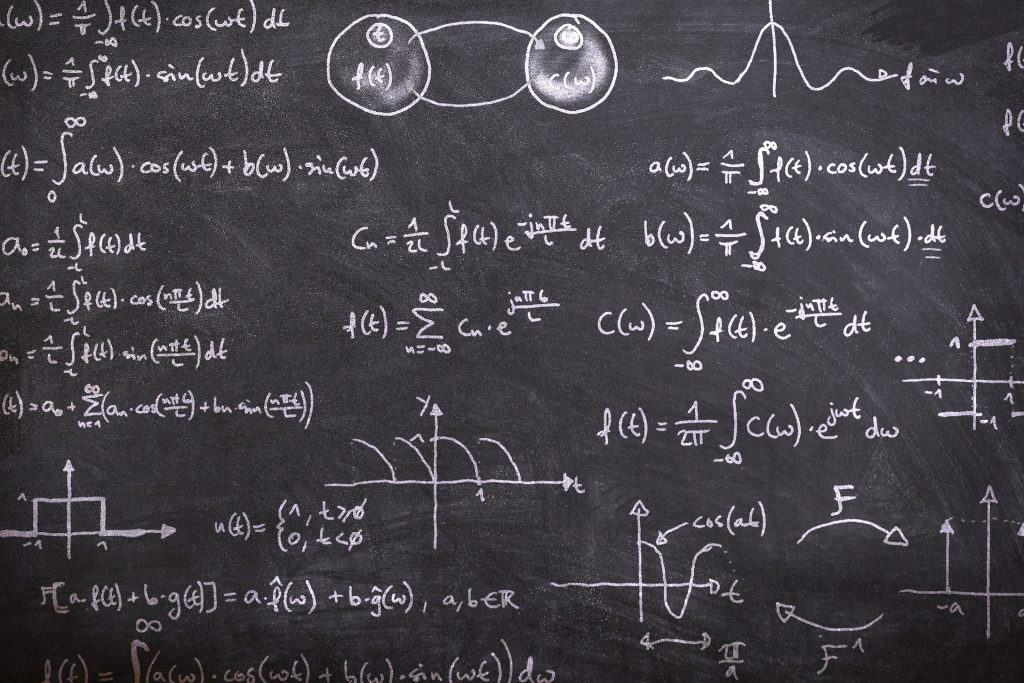# 6) H2 Mathematics (yearly access)

Current Status
Not Enrolled
Price
Closed
Get Started
This course is currently closed

Welcome.

## Course Description

In this h2 mathematics course, you will be learning

1. Basic Properties of Vectors
2. Scalar and Vector Products in Vectors
3. Equation of lines and planes
4. Three Dimensional Geometry
5. Functions
6. Graphs and Transformations
7. Equations and Inequalities
8. Sequence and Series
9. Complex Number expressed in cartesian form
10. Complex Number expressed in polar form
11. Differentiation
12. Application of Differentiation
13. Maclaurin Series
14. Integration Techniques
15. Application of Integration
16. Differential Equations
17. Permutations and Combinations
18. Probability
19. Discrete Random Variables
20. Binomial Distribution
21. Normal Distribution
22. Sampling
23. Hypothesis Testing
24. Correlation and Linear Regression

## Course Content

Lesson Content
0% Complete 0/7 Steps
Lesson Content
0% Complete 0/8 Steps
Lesson Content
0% Complete 0/4 Steps
Lesson Content
0% Complete 0/14 Steps
Lesson Content
0% Complete 0/7 Steps
Lesson Content
0% Complete 0/7 Steps
Lesson Content
0% Complete 0/6 Steps
Lesson Content
0% Complete 0/7 Steps
Lesson Content
0% Complete 0/7 Steps
Lesson Content
0% Complete 0/7 Steps
Lesson Content
0% Complete 0/8 Steps
Lesson Content
0% Complete 0/5 Steps
Lesson Content
0% Complete 0/9 Steps
Lesson Content
0% Complete 0/8 Steps
Lesson Content
0% Complete 0/3 Steps
Lesson Content
0% Complete 0/4 Steps
Lesson Content
0% Complete 0/6 Steps
Lesson Content
0% Complete 0/9 Steps
Lesson Content
0% Complete 0/6 Steps
Lesson Content
0% Complete 0/3 Steps
Lesson Content
0% Complete 0/10 Steps
Lesson Content
0% Complete 0/10 Steps
Lesson Content
0% Complete 0/8 Steps
Lesson Content
0% Complete 0/9 Steps